Linear Quadratic Systems (line-parabola) Terms of Use    Contact Person: Donna RobertsDirections: Grab paper, pencil, and a stratight-edge. You may also want a sheet of graph paper. Use your graphing calculator only for checking your results. Beware!! The cartoon assistants may, or may not, be helpful!

 1. Solve and check this system algebraically:         y = x2 - x - 6      y = x - 3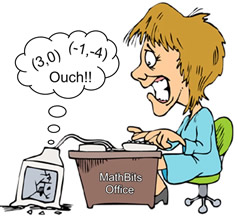2. Solve and check this system algebraically:          2x + y = 2       y = (x - 1)2 + 13. Solve and check this system by graphing:         y = x + 3      y = x2 - 2x - 1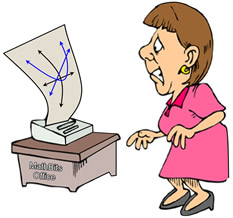4. Solve and check this system by graphing:              y = -x2 - 2x + 8           2x - y = -3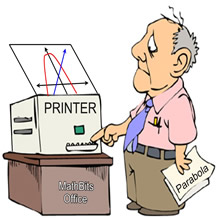5.
Which ordered pair is a solution to the system of equations:
y = x2 - x    and    y = x + 3 ?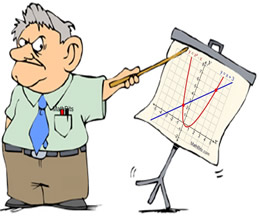Choose:
 (2,5) (3,-1) (3,6) (6,9)

6.
How many unique solutions are there to the following system of equations?
y = x2 - 5x + 3    and    y = x - 6Choose:
 1 2 3 0

7.
Which ordered pair is a solution to the system of equations graphed at the right?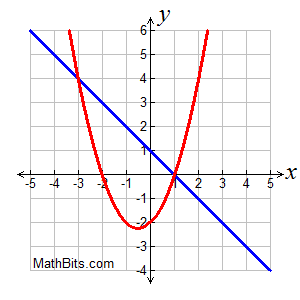Choose:
 (-3,1) (-3,4) (1,1) (0,1)

8.
How many unique solutions are there to the following system of equations?
y = -x2 - x + 2    and    x + 3y = 12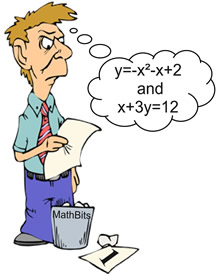Choose:
 1 2 3 0